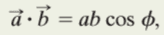# Component of a Vector

Foehammer

## Homework Statement

[/B]
Here are two displacements, each measured in meters:
A. 4i+5j-6k
B. -1i+2j+3k

What is the component of A that is perpendicular to the direction of B and in the plane of A and B.?

## Homework Equations

The book gave me a hint to use this equation:

http://puu.sh/fFya9/3d102e2d2f.png [Broken]

c being the magnitude of the result of A cross B. a and b being the magnitudes of vectors A and B.

## The Attempt at a Solution

[/B]
I plugged the values into that:

c=(8.775)*(3.742)*sin(111.438)
c=30.564

I don't see how that is supposed to help me.

A cross B gives me the vector:

C=27i-6j+13k

This vector is perpendicular to the A,B plane.

Would I need to cross C by B again to get it back in the A,B plane? After doing that would I need to use this equation to find the final component part?Doing that gives me:

D=-44i-94j+48k

A dot D=-934

-934=8.775*114.35*cos(phi)

phi=158.56

I think this is the angle between vectors A and D. Then is it just a*cos(158.56)

8.77*cos(158.56)=-8.17

I'm not 100% sure that is correct as I feel I messed up with being able to cross product twice to get back on the A,B plane. Your help is greatly appreciated.

Last edited by a moderator:

Homework Helper
Gold Member
You are on the right track (I didn't check your numbers). Perhaps it is simpler to consider this: once you have vector D = B x C, find a unit vector in the direction of D. Then take the dot product of this unit vector with A. To find a unit vector in the direction of D, just divide vector D by its magnitude.

Foehammer
Alright here it is:

B x C=D=44i+94j-48k

D as a unit vector is:

.3849i+.8221j-.4198k

A dot D=8.169

8.169=8.775*1*cos(phi)

phi=21.418

Using this new angle it comes out to be:

8.77*cos(21.418)=8.164

So, one thing I noticed was that in my original post I did C x B and you recommended B x C. I know that the order of cross product does matter so why did you choose the order you did? I also see that finding the dot product between the two was my answer and I didn't need to use the cos(phi) formula part. So, I think my final answer is 8.164? It seems more reasonable since it isn't negative.

Homework Helper
Gold Member
You don't need to calculate new angle phi. The dot product of A with a unit vector in the direction of D is the component of A in the direction of D which is what the problem asks for. Note how close your answer A dot D = 8.169 is to your final answer 8.164. The difference is round offs and you went around in a circle when you calculated that last bit.

As to why I chose B x C as opposed to C x B, the answer is that for this problem it doesn't matter. One is the negative of the other. Draw a drawing and you will see that there are two perpendiculars "to the direction of B and in the plane of A and B" as the problem specifies. One is the negative of the other. In your original post, you calculated the other solution. Note that the sum of your original angle and this angle are 158.56 + 21.418 = 180 as they should. Both of these angles are correct and the component that you are seeking is either 8.169 or -8.169 depending on which of the two perpendiculars "to the direction of B and in the plane of A and B" you choose.

Last edited:
Foehammer
You don't need to calculate new angle phi. The dot product of A with a unit vector in the direction of D is the component of A in the direction of D which is what the problem asks for. Note how close your answer A dot D = 8.169 is to your final answer 8.164. The difference is round offs and you went around in a circle when you calculated that last bit.

As to why I chose B x C as opposed to C x B, the answer is that for this problem it doesn't matter. One is the negative of the other. Draw a drawing and you will see that there are two perpendiculars "to the direction of B and in the plane of A and B" as the problem specifies. One is the negative of the other. In your original post, you calculated the other solution. Note that the sum of your original angle and this angle are 158.56 + 21.418 = 180 as they should. Both of these angles are correct and the component that you are seeking is either 8.169 or -8.169 depending on which of the two perpendiculars "to the direction of B and in the plane of A and B" you choose.

AAhh alright. Thank you for clearing that up. I see the relationship now and it makes sense. Thanks again!

azizlwl
What is the component of A that is perpendicular to the direction of B .

Magnitude A x Sine θ, where θ between A and B.
ASineθ =A1Sineθ=vectot A cross unit vector B which is a vector.